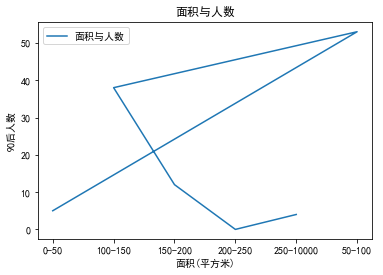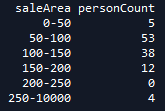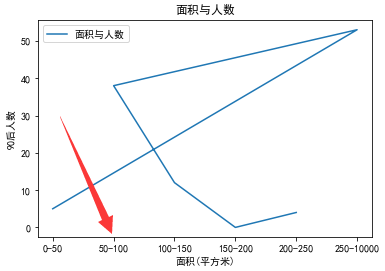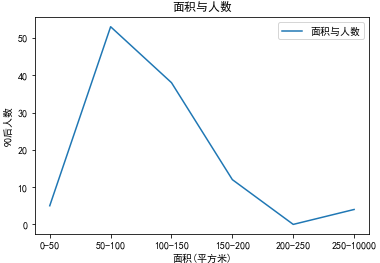• 主要介绍了详解Python Matplotlib解决绘图X轴值不按数组排序问题，文中通过示例代码介绍的非常详细，对大家的学习或者工作具有一定的参考学习价值，需要的朋友们下面随着小编来一起学习学习吧
• #coding=utf-8 #Program python3.6 import pandas as pd # 数据分析 import os # 操作系统OS import matplotlib.pyplot as plt # 可视化...
#coding=utf-8
#Program python3.6

import pandas as pd                             # 数据分析
import os                                       # 操作系统OS
import matplotlib.pyplot as plt                 # 可视化图表
import numpy as np                              # 科学计算
from dateutil.parser import parse               # 日期时间
import  warnings                                # 警报处理
warnings.filterwarnings("ignore", module="matplotlib") # 忽略matplotlib警告信息

print('程序功能：根据起止日期，收集研磨组制程检查记录数据，D:\文件夹下生成XBar-R图pdf文档')

mydate = '2019-11-1,2019-11-30'
date_low = parse(mydate.split(','))
date_upper = parse(mydate.split(','))
drawing = '1101-1070276'

xls = pd.ExcelFile(r'd:\python\研磨各工序首末件.xlsm')                              # 读取excel数据
source.drop([1,3,4,5,6,8],axis=1,inplace=True)                  # 删除不需要的列
source.drop(source[source!=drawing].index,inplace=True)      # 删除A列不等于drawing所在行
source.dropna(how='any')                                        # 删除空值
source.drop(source[source == '检查频率/时间'].index,inplace=True) # 清除“检查频率/时间”所在行
source = source[(source.iloc[:,1]>=date_low) & (source.iloc[:,1]<=date_upper)]  # 按日期截取数据
gp = source.groupby(by=,sort = True)                         # 按检查内容分组
length = len(gp)                                                # 检查内容项目数量  #source.set_index(,inplace=True,drop=False)
i=0
fig,ax = plt.subplots(nrows=length,ncols=1,sharex=True,figsize=(11.5,8.2))         # 定义子图数量，共用X轴坐标
ax.set_title('{} Xbar-R控制图'.format(drawing),fontsize=18)  # 定义标题

for k,v in gp:
ar = []
v = v.dt.strftime('%m-%d')
v.set_index(,inplace=True)

for x in v.values:   # 这里用法很有问题
ar.extend(x[2:])

ax[i].plot(ar,linestyle='--',linewidth=1.1,marker='.',markersize=6)
ax[i].get_yaxis().get_major_formatter().set_useOffset(False)   # 纵轴刻度由科学计数法改为标准数学法
ax[i].set_xlabel(k.replace('\n',''),weight='normal',fontsize=12)
ax[i].grid(True,alpha=0.6)
i=i+1

plt.rcParams['font.sans-serif'] = ['SimHei']                    # 中文支持  #plt.rcParams['font.size']=8
plt.tight_layout()
plt.subplots_adjust(left=0.06, bottom=0.07, right=0.97,top=0.94,hspace=0.2, wspace=0.15)  ## 调整边距，子图间距
plt.savefig(r'd:\python\{}.pdf'.format(drawing))                # 存为pdf文档
plt.show()                                                      # 图形显示

展开全文pandas matplotlib
• Python+Matplotlib解决X 轴值不按数组排序最简单的办法问题描述解决方案复制害死人最终解决 问题描述 看标题就知道了，先上个图给大家 这个图对应的代码和数据如下，也是网上找到的最初的代码，根据自己的数据进行...

问题描述

看标题就知道了，先上个图给大家这个图对应的代码和数据如下，也是网上找到的最初的代码，根据自己的数据进行了略微改动，也是最简单的办法，让你会感觉plt的使用简直是太简单了。# 折线图
plt.rcParams['font.sans-serif'] = ['SimHei']  # 用来正常显示中文标签
plt.rcParams['axes.unicode_minus'] = False  # 用来正常显示负号
x=dfGraph['saleArea']
y1=dfGraph['personCount']
plt.plot(x,y1,label='面积与人数')

plt.xlabel('面积(平方米)')
plt.ylabel('90后人数')
plt.title('面积与人数')
plt.legend()
plt.show()

解决方案

上图中，问题在于X轴的数据顺序不对，原因是plt在显示数据的时候，自己按照顺序进行重新排序，结果造成“50-100”这个数值排到了最后，造成了图形很奇怪。有一个办法就是把X轴的数值定义按照ASCII的顺序，但是由于X轴数据是自动动态读取的，靠手工是不可靠的。
经过几度查询，找到以下这篇文章：
Python之Matplotlib 解决绘图 X 轴值不按数组排序问题

这篇文章写的还是不错，至少知道了思路，但是这篇文章有一点不明白，就是这句：

# 自定义 x轴 的取值：
plt.xticks(arange(len(colums_x)),colums_x)

用了以后语法始终报错，说没有定义，网上竟然没有找到类似的问题，难道是我的python版本问题吗？

复制害死人

有时候，复制粘贴的便利性造成了程序员的不动脑筋，上面说的那篇文章，在各个地方都能搜到，也不知道到底哪个网站的才是原创！问题关键是用的都是arange!
后来阴差阳错的把arange改成了range，语法竟然不报错了，立马测试了一下，大家先看一下效果：最终解决

看上图，终于解决了X轴排序的问题，但是为啥图形还是那么奇怪呢？聪明的读者大概猜到是什么问题了，上图图形对应的代码如下：

plt.xticks(range(len(x)),x)
plt.plot(x,y1,label='面积与人数')

结合之前那篇文章，问题一下子就找到了，是第二句的问题，最终正确的代码和图形如下：

plt.xticks(range(len(x)),x)
plt.plot(y1,label='面积与人数')

这下图形终于对了，又学到了新知识！多说一句，50-100平方米的购买人数果然多啊。

展开全文python matplotlib
• 主要介绍了Python设置matplotlib.plot的坐标刻度间隔以及刻度范围，具有一定的参考价值，感兴趣的小伙伴们可以参考一下
• 我有两个列表,我正在使用ipython笔记本中...它应该从星期六开始,这是我的date_list中的第一个值.(忽略这里的y轴值,我刚刚为这个例子做了count_list值.)import numpy as npimport matplotlib.pyplot as plt%matplotl...

我有两个列表,我正在使用i

python笔记本中的基本折线图.应该是基本的,但我不断像这样搞砸x轴…它应该从星期六开始.

我的代码有什么问题让我的x轴标签搞砸了？它应该从星期六开始,这是我的date_list中的第一个值.

(忽略这里的y轴值,我刚刚为这个例子做了count_list值.)

import numpy as np

import matplotlib.pyplot as plt

%matplotlib inline

count_list = [5,7,2,3,1,7,3]

date_list = ['Saturday', 'Sunday', 'Monday', 'Tuesday', 'Wednesday', 'Thursday', 'Friday']

fig=plt.figure(figsize=(12,3.5))

ticknumbs = np.arange(7)

ax.plot(ticknumbs, count_list, color='r', linewidth=1.0)

ax.set_xticklabels(date_list, rotation = 45) #WHHHHY ARENT U WORKING

ax.margins(x=0.05, y=0.05)

展开全文• 我正在从一个简单的sqlite3 DB中查询数据，该数据库正在提取系统...到目前为止，我使用以下代码：import matplotlib as mplmpl.use('Agg') # force no x11import matplotlib.pyplot as pltimport sqlite3con = sqli...

我正在从一个简单的sqlite3 DB中查询数据，该数据库正在提取系统上观察到的每个端口的连接数列表。我试着用matplotlib把它画成一个简单的条形图。

到目前为止，我使用以下代码：import matplotlib as mpl

mpl.use('Agg') # force no x11

import matplotlib.pyplot as plt

import sqlite3

con = sqlite3.connect('test.db')

cur = con.cursor()

cur.execute('''

SELECT dst_port, count(dst_port) as count from logs

where dst_port != 0

group by dst_port

order by count desc;

'''

)

data = cur.fetchall()

dst_ports, dst_port_count = zip(*data)

#dst_ports = [22, 53223, 40959, 80, 3389, 23, 443, 35829, 8080, 4899, 21320, 445, 3128, 44783, 4491, 9981, 8001, 21, 1080, 8081, 3306, 8002, 8090]

#dst_port_count = [5005, 145, 117, 41, 34, 21, 17, 16, 15, 11, 11, 8, 8, 8, 6, 6, 4, 3, 3, 3, 1, 1, 1]

print dst_ports

print dst_port_count

fig = plt.figure()

# aesthetics and data

plt.grid()

plt.bar(dst_ports, dst_port_count, align='center')

#plt.xticks(dst_ports)

# labels

plt.title('Number of connections to port')

plt.xlabel('Destination Port')

plt.ylabel('Connection Attempts')

# save figure

fig.savefig('temp.png')

当我运行上面的代码时，成功地从数据库中检索到数据并生成一个图。然而，这张图并不是我所期望的。例如，在x轴上，它绘制0到5005之间的所有值。我希望它只显示dst_端口中的值。我试过使用xticks，但这也不起作用。

我在上面的代码中包含了一些示例数据，我已经注释掉了这些数据，这些数据可能很有用。

此外，下面是上述代码的图形输出示例：使用xticks时也会出现一个grpah：展开全文• matplotlib绘图X轴标签不完全显示解决方法 举例： 现从excel中读取日期为X轴X轴的刻度设置为每10个日期为一个间隔，但是绘图X轴的标签没有对应上刻度显示，如下图所示 主要代码如下： import pandas as pd import...python
• class matplotlib.ticker.ScalarFormatter(useOffset=True,useMathText=False, useLocale=None) Bases: matplotlib.ticker.FormatterTick location is a plain old number. If useOffset==True and the datarange is...
• 很久没有在CSDN写博客了，非常地惭愧，在工作、...今天遇到的问题为，在jupyter notebook上用matplotlib画一组与时间有关的数据，结果X轴的时间显示出现了点问题，主要代码片如下： #画时间曲线的函数 def plot_cu...python 数据分析
• 二、借助matplotlib.ticker.FuncFormatter()，将坐标格式化。 例子： # encoding=utf-8 import matplotlib.pyplot as plt from matplotlib.ticker import FuncFormatter plt.rcParams['font.family'] = ['Times ...
• import matplotlib as mt import numpy as np y=[7,0,0,0,0,0,1,25,98,333,471,0,322,429,425,478,385,237,219,284,351,364,165,0] x=[1,2,3,4,5,6,7,8,9,10,11,12,13,14,15,16,17,18,19,20,21,22,23,24] x1=np....
• 加上这一行 plt.xticks(rotation=270) 效果如图python
• import matplotlib.pyplot as plt ''' numpy.linspace(start, stop, num=50, endpoint=True, retstep=False, dtype=None): start就是开始的数字 stop就是结束的数字 num就是返回的数量 endpoint是一个bool型变量，...python matplotlib
• 简单描述就是，首先拿到的是时间戳与每个时间戳对应的 value ，需要绘制折线图，并且，x 需要按照类似于上面图片所示的格式。 例子代码如下： import matplotlib.pyplot as plt import matplotlib.dates as md ...
• 我正试图对数据进行一些分析。我得到csv文件并将其转换为pandas...它有好几列，但我试图将x轴绘制为日期列。熊猫数据框如下print (df.head(10)cus-id date value_limit0 10173 2011-06-12 4551 95062 2011-09-11 ...
• 更改x轴刻度显示内容：x轴要显示为如下name中的标签；同时刻度要旋转30度；注意name为一个list names=['0-20','20-40','40-60','60-80','80-100','100-120','120-140','140-160','160-180','180-200','200-220','...
• 解决方法一（每次新建项目均需操作一遍，较麻烦）： ... （1）下载SimHei.ttf字体文件，双击安装 ...（2）将上述字体文件拷贝到matplotlib字体文件夹~/virtualenvs/~/lib/python3.7/site-packages/mat...
• 在机器学习中经常会使用Sigmoid函数，如果直接使用matplotlib绘图，那么就会像下图这样，原点并没有在(0,0)。 import matplotlib.pyplot as plt ...于是，我们希望调整坐标的位置，让图像更加的直观
• import matplotlib.pyplot as plt fig = plt.figure(figsize=(20, 5)) ax = fig.add_subplot(111) ax.plot(single_data['2015-01-01':'2015-12-31']) ax.plot(single_data['2016-01-01':'2016-12-31']) plt....python 可视化
• 1) bar中的两个参数： left：柱形的左边缘的位置，如果我们指定1那么当前柱形的左边缘的x就是1.0了 height：这是柱形的高度，也就是Y轴的了 plt.xticks 就是每个a对应的label_list，这个是为了画出x轴的标签。...
• matplotlib绘制x轴为时间的图

万次阅读 2018-06-25 19:51:42
图形可视化中时间应该是比较常见的了，不管在R语言中还是Python中，都可以直接plot（x，y）直接绘制出来，但很大概率坐标文字重叠，因为将时间直接当做字符串处理，若还想自定义时间格式，必须先将数据转换为...
• 有一个网友碰到这样一个问题，如果直接使用%H来设置...深入浅出matplotlib(35)：设置坐标个性化显示标签 https://mysoft.blog.csdn.net/article/details/111479756 在这里演示的例子如下： #深入浅出matplot.python matplotlib
• python使用matplotlib画图时设置x轴刻度 plt.rcParams['font.family'] = ['KaiTi'] plt.rcParams['axes.unicode_minus'] = False %matplotlib inline figure = plt.figure(figsize=(26,8)) ax1 = figure.add_...python 机器学习 数据挖掘
• 在使用matplotlib绘制图片时，x轴的刻度可能比较密集，特别是以日期作为x轴时，则最后会显示不出来。 数据如下： 绘制随时间变化的的折线图。 直接绘制折线图，可以发现x轴重叠。 plt.plot(Date, V1, 'r', label='...python matplotlib
• 我用下面的代码将数据绘制成一个散点图，并对不同的子图使用相同的。在import numpy as npimport matplotlib.pyplot as pltepsilon= np.array([1,2,3,4,5])f, (ax1, ax2, ax3, ax4) = plt.subplots(4, sharex= ...
• 我试图使图形线条平滑,但是由于x轴值是日期,因此这样做非常麻烦.说我们有一个数据框如下import matplotlib.pyplot as pltimport numpy as npimport pandas as pd%matplotlib inlinestartDate = '2015-05-15'endDate ...
• plt.xticks(rotation=90) # x轴标签竖着显示；90为标签逆时针旋转度数 plt.yticks(rotation=90) # y轴标签横着显示；90为标签逆时针旋转度数 也可以自动旋转x轴标签： fig.autofmt_xdate() ...css 前端...